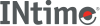﻿ rintINtime SDK Help
rint

Round-to-nearest integral value.

```#include <math.h>

double rint(double x);
```

#### Parameters

`x`
A number of type Double or any valid numeric expression.

#### Remarks

These functions shall return the integral value (represented as a double) nearest `x` in the direction of the current rounding mode. The current rounding mode is implementation-defined.

If the current rounding mode rounds toward negative infinity, then rint shall be equivalent to floor. If the current rounding mode rounds toward positive infinity, then rint shall be equivalent to ceil.

#### Return Values

Upon successful completion, these functions shall return the integer (represented as a double precision number) nearest `x` in the direction of the current rounding mode.

If `x` is NaN, a NaN shall be returned.

If `x` is +/-0 or +/-Inf, `x` shall be returned.

If the correct value would cause overflow, a range error shall occur and rint, rintf, and rint shall return the value of the macro +/-HUGE_VAL, +/-HUGE_VALF, and +/-HUGE_VALL (with the same sign as `x`), respectively.

#### Requirements

Versions Defined in Include Link to
INtime 3.0 intime/rt/include/math.h math.h clib.lib